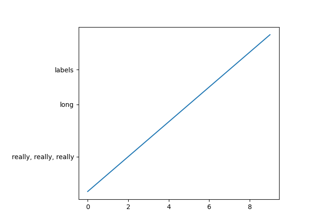# matplotlib.figure.SubplotParams¶

class matplotlib.figure.SubplotParams(left=None, bottom=None, right=None, top=None, wspace=None, hspace=None)[source]

Bases: object

A class to hold the parameters for a subplot.

Defaults are given by rcParams["figure.subplot.[name]"].

Parameters: leftfloatThe position of the left edge of the subplots, as a fraction of the figure width. rightfloatThe position of the right edge of the subplots, as a fraction of the figure width. bottomfloatThe position of the bottom edge of the subplots, as a fraction of the figure height. topfloatThe position of the top edge of the subplots, as a fraction of the figure height. wspacefloatThe width of the padding between subplots, as a fraction of the average axes width. hspacefloatThe height of the padding between subplots, as a fraction of the average axes height.
__dict__ = mappingproxy({'__module__': 'matplotlib.figure', '__doc__': '\n A class to hold the parameters for a subplot.\n ', '__init__': <function SubplotParams.__init__>, 'update': <function SubplotParams.update>, '__dict__': <attribute '__dict__' of 'SubplotParams' objects>, '__weakref__': <attribute '__weakref__' of 'SubplotParams' objects>, '__slotnames__': []})
__init__(self, left=None, bottom=None, right=None, top=None, wspace=None, hspace=None)[source]

Defaults are given by rcParams["figure.subplot.[name]"].

Parameters: leftfloatThe position of the left edge of the subplots, as a fraction of the figure width. rightfloatThe position of the right edge of the subplots, as a fraction of the figure width. bottomfloatThe position of the bottom edge of the subplots, as a fraction of the figure height. topfloatThe position of the top edge of the subplots, as a fraction of the figure height. wspacefloatThe width of the padding between subplots, as a fraction of the average axes width. hspacefloatThe height of the padding between subplots, as a fraction of the average axes height.
__module__ = 'matplotlib.figure'
__slotnames__ = []
__weakref__

list of weak references to the object (if defined)

update(self, left=None, bottom=None, right=None, top=None, wspace=None, hspace=None)[source]

Update the dimensions of the passed parameters. None means unchanged.

## Examples using matplotlib.figure.SubplotParams¶